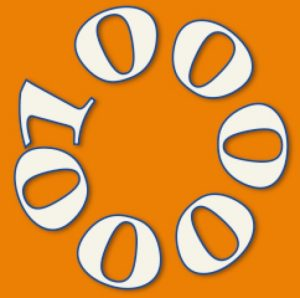Easy starter:  Write down 2 numbers that add up to a million, one smaller than           450 000 and one bigger than 450 000.If you shared a prize of one million dollars between 7 people how many dollars would each get?

What is the easiest way to work out which of the following is divisible by 7?

(a) one million minus one
(b) one million minus two
(c) one million minus three
(d) one million minus four
(e) one million minus five

How would you work out which is divisible by 6?

What are the prime factors of one million?

Tagged with: